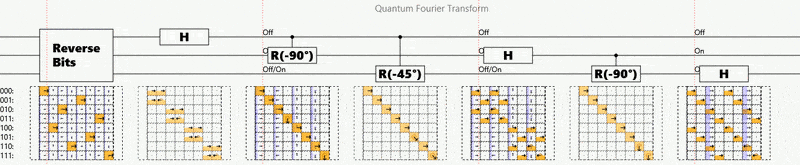# Quantum computing

MasterSashin04
Hi i just wanted to know what the main difference between quantum computers and normal computers are?
Is it that a Qubit can be 1 and 0 at the same time... becaus that is what i found after i researched however my friend states that the quantum computer can run many different sets of code at the same time instead of one. Or can work with an infinite amount of binary code.

## Answers and Replies

Phys&Psych
It isn't just that a qubit can be 1 and 0 simultaneously; it can simultaneously be everything between those 2 values. This theroretically allows for computations to be performed many, many times faster than current computers. The current iteration of the quantum computer (I am only aware of one at this time) may not actually be a quantum computer. One problem is the designers and builders aren't totally sure how it works. They only know it can perform certain computations much faster than conventional devices. It would be useless on your desktop.

If you already know some computer science, then this post about Grover's algorithm might clarify what's going on a bit.

Basically quantum mechanics allows superpositions of classical states, meaning you get to assign a complex weight to every possible state instead of only being in one. Quantum mechanics also allows you to apply matrix operations to these large vectors of state weightings. So you get to do huge matrix multiplications very cheaply, but you have to deal with the fact that you only get to sample from the results (i.e. the superposition collapses when you measure the state) and that the type of matrix you can use is quite restrictive (i.e. must be reversible and must preserve total probability in all cases).

Although it might not be so clear on its own, here's an animation of the Quantum Fourier Transform (which uses only ~log(N)^2 gates to Fourier transform N amplitudes and is a crucial part of Shor's factoring algorithm):Abhishek Sethi
Hi,
In any form of computation, there is a physical construct behind it. The fundamental difference between quantum computing and classical computing is due to the different structure used. For example, in classical computing, a bit can be represented by a transistor. Physical quantities(like electric field, current density etc) associated with the transistor determine the value of this bit. So, the value of the bit represents some physical property. The value of the bit can be changed by manipulating the system physically, maybe by applying an external magnetic field. So, the operator or "a logic gate" represents a physical manipulation. The limitations and the capabilities of the physical construct is what determines the limitations and capabilities of the computation.
You would need to understand QM in order to understand the representation of a bit(qubit) and the operations that can be used(operators). This is what makes quantum computing different from classical computing.
I can point some features of QM that makes QC what it is,
1) Principle of superposition of multiple wave functions
2) Correlation between multiple bits(qubits) or quantum entanglement

Some popular advantages in QC over CC,
1) Grovers search algorithm(faster than binary search algorithm)
2) Shors Algorithm to find prime factors(works great under some constraints)

Correct me if I am wrong somewhere.
Hope it helps.